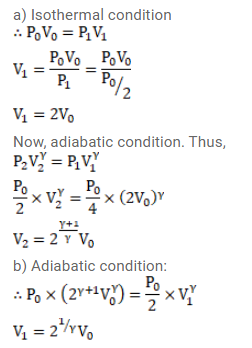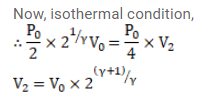# Consider a given sample of an ideal gas (Cp/Cv=γ)`
Question:

Consider a given sample of an ideal gas $\left(C_{p} / C_{v}=\gamma\right)$ having initial pressure $p_{0}$ and volume $V_{0}$. (a) The gas is isothermally taken to a pressure $\mathrm{p}_{0} / 2$ and from there adiabatically to a pressure $\mathrm{p}_{0} / 4$. Find the final volume. (b) The gas is brought back to its initial state. It is adiabatically taken to a pressure $p_{0} / 2$ and from there isothermally to a pressure $p_{0} / 4$. Find the final volume.

Solution: